The LOGISTIC Procedure
 Overdispersion

For a correctly specified model, the Pearson chi-square statistic and the deviance, divided by their degrees of freedom, should be approximately equal to one. When their values are much larger than one, the assumption of binomial variability might not be valid and the data are said to exhibit overdispersion. Underdispersion, which results in the ratios being less than one, occurs less often in practice.

When fitting a model, there are several problems that can cause the goodness-of-fit statistics to exceed their degrees of freedom. Among these are such problems as outliers in the data, using the wrong link function, omitting important terms from the model, and needing to transform some predictors. These problems should be eliminated before proceeding to use the following methods to correct for overdispersion.

### Rescaling the Covariance Matrix

One way of correcting overdispersion is to multiply the covariance matrix by a dispersion parameter. This method assumes that the sample sizes in each subpopulation are approximately equal. You can supply the value of the dispersion parameter directly, or you can estimate the dispersion parameter based on either the Pearson chi-square statistic or the deviance for the fitted model.

The Pearson chi-square statistic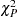and the deviance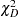are given by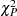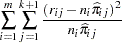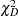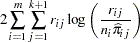whereis the number of subpopulation profiles,is the number of response levels,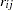is the total weight (sum of the product of the frequencies and the weights) associated withth level responses in theth profile,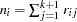, and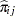is the fitted probability for theth level at theth profile. Each of these chi-square statistics has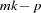degrees of freedom, where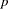is the number of parameters estimated. The dispersion parameter is estimated by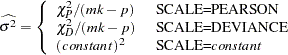In order for the Pearson statistic and the deviance to be distributed as chi-square, there must be sufficient replication within the subpopulations. When this is not true, the data are sparse, and the p-values for these statistics are not valid and should be ignored. Similarly, these statistics, divided by their degrees of freedom, cannot serve as indicators of overdispersion. A large difference between the Pearson statistic and the deviance provides some evidence that the data are too sparse to use either statistic.

You can use the AGGREGATE (or AGGREGATE=) option to define the subpopulation profiles. If you do not specify this option, each observation is regarded as coming from a separate subpopulation. For events/trials syntax, each observation representsBernoulli trials, whereis the value of the trials variable; for single-trial syntax, each observation represents a single trial. Without the AGGREGATE (or AGGREGATE=) option, the Pearson chi-square statistic and the deviance are calculated only for events/trials syntax.

Note that the parameter estimates are not changed by this method. However, their standard errors are adjusted for overdispersion, affecting their significance tests.

### Williams’ Method

Suppose that the data consist ofbinomial observations. For theth observation, let
be the observed proportion and let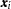be the associated vector of explanatory variables. Suppose that the response probability for theth observation is a random variable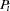with mean and variance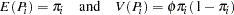where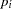is the probability of the event, and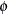is a nonnegative but otherwise unknown scale parameter. Then the mean and variance of
are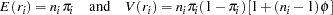Williams (1982) estimates the unknown parameterby equating the value of Pearson’s chi-square statistic for the full model to its approximate expected value. Suppose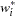is the weight associated with theth observation. The Pearson chi-square statistic is given by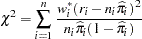Let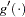be the first derivative of the link function. The approximate expected value of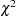is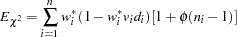where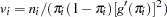and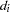is the variance of the linear predictor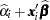. The scale parameteris estimated by the following iterative procedure.

At the start, let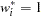and let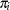be approximated by,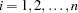. If you apply these weights and approximated probabilities toand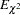and then equate them, an initial estimate ofis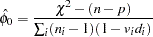whereis the total number of parameters. The initial estimates of the weights become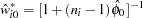. After a weighted fit of the model, the
and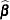are recalculated, and so is. Then a revised estimate ofis given by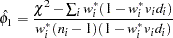The iterative procedure is repeated untilis very close to its degrees of freedom.

Oncehas been estimated by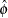under the full model, weights of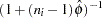can be used to fit models that have fewer terms than the full model. See Example 51.10 for an illustration.

Note:If the WEIGHT statement is specified with the NORMALIZE option, then the initialvalues are set to the normalized weights, and the weights resulting from Williams’ method will not add up to the actual sample size. However, the estimated covariance matrix of the parameter estimates remains invariant to the scale of the WEIGHT variable.Previous Page | Next Page | Top of Page